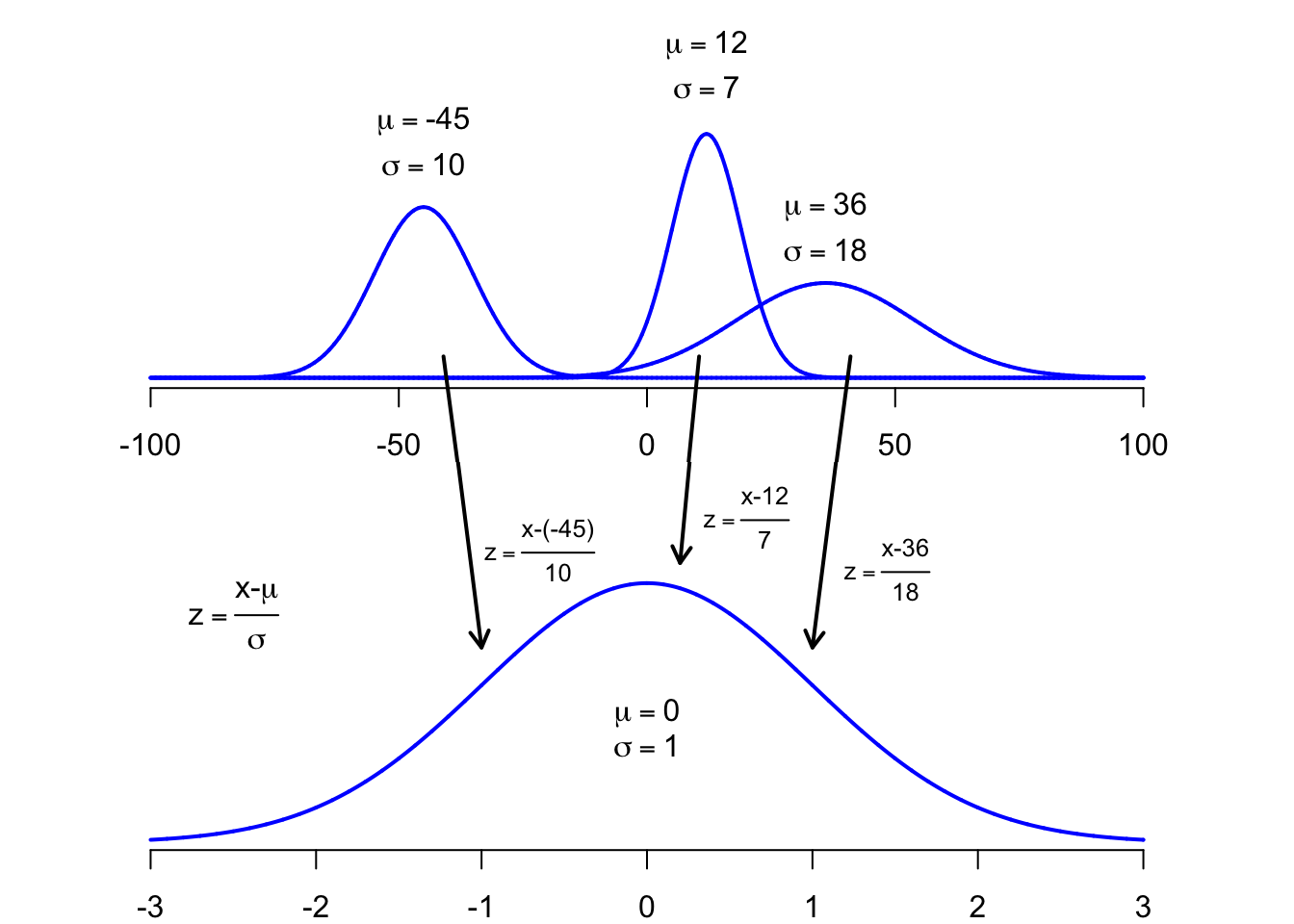Before we can apply the concept of a standard normal distribution to a real data set we need to discuss the concept of standardization of a normal distribution. We know that a normal distribution is parametrized by two parameters, its mean $$\mu \in \mathbb R$$ and its standard deviation $$\sigma \in \mathbb R>0$$, $$X \sim N(\mu,\sigma)$$. The actual value of these parameters depends on the population and the metrics used to describe its features. In order to transform any particular $$\mu$$ and $$\sigma$$, related to a particular random variable $$X$$, to $$\mu=0$$ and $$\sigma=1$$, we have to convert the $$x$$-value to a $$z$$-value, by applying the equation below.

$z = \frac{x-\mu}{\sigma}$

As a result we get a standard normal distribution for any particular normal distribution. This procedure is essential if you need to determine the $$z$$-scores or any particular probability related to a $$z$$-score $$(P(z))$$ by looking them up in a table. We will see later on, that R is such a powerful tool, which makes the step of standardization dispensable.